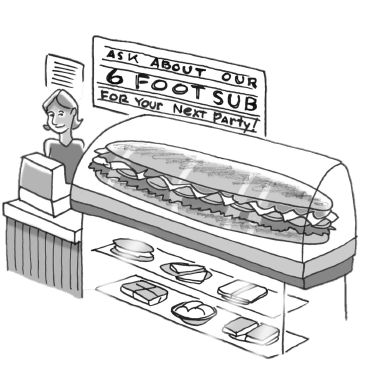### Home > CC3MN > Chapter 7 > Lesson 7.2.4 > Problem7-63

7-63.Salami and More Deli sells a $6$-foot sandwich for parties. It weighs $8$ pounds. Assume the weight per foot is constant.

1. How much does a sandwich $0$ feet long weigh?

$0$ pounds

1. Draw a graph showing the weight of the sandwich (vertical axis) compared to the length of the sandwich (horizontal axis). Label the axes with appropriate units.

Graph starting with the point where $x=0$

Your graph should show a line with positive slope.
Units on the vertical axis should be in pounds.
Units on horizontal axis should be in feet.

1. Use your graph to estimate the weight of a $1$-foot sandwich.

What is the value of $y$ when $x=1$?

2. Write a proportion to find the length of a $12$-pound sandwich.

Compare sandwich length and height.

$\frac{6}{8}=\frac{\it x}{12}$

$9$ feet